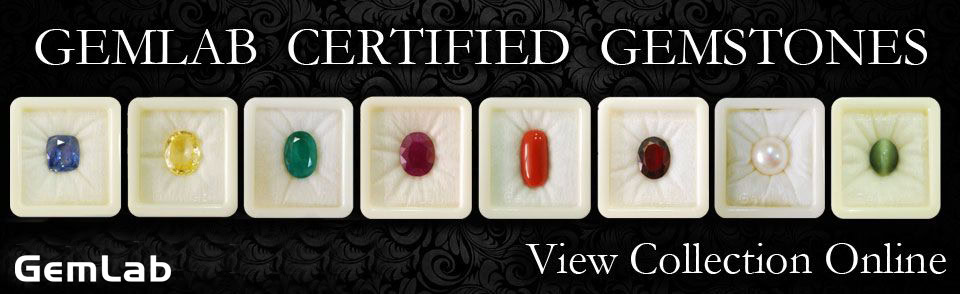# Basic Crystallography

All gemstones can be classified as either:

### Amorphous

1. No orderly internal atomic structure.
2. No naturally-occurring characteristic shape.
3. Products of rapid cooling.
4. Physical properties are constant in all directions.

### Crystalline

1. Definite & regular internal atomic structure.
2. Geometrical external forms.
3. Directional properties.
4. Products of slow cooling.
5. Identical in all crystals of a given species.

Gemstones can also be:

### Massive

A term used to describe a crystalline substance that does not have a naturally-occurring characteristic shape. (i.e. Rose Quartz)

### Crypto-crystalline

This type of material has not grown as a single crystal but as an aggregate of small or microscopic crystals randomly orientated within the body of the gem.

These gems are usually semi-translucent or opaque and slightly porous. Although all crypto-crystalline gems are massive, it should be noted that not all massive materials are crypto-crystalline. (i.e. Chalcedony Quartz)

### Habit

The characteristic crystal form displayed by a mineral.

## Crystallography

Crystallography is defined as the study of crystals and their structure.

There are 3 elements of symmetry that are fundamental to the study of crystallography.

Symmetry: correspondence, the similarity in or between the opposing sides of an object, considered as halves on either side of a central line, in form and dimensions.

Axis of Symmetry: an imaginary line passing through a crystal that will permit a characteristic representation of a crystal 2, 3, 4 or 6 times during each 360-degree rotation.

The plane of Symmetry: this is a plane through a crystal which divides the crystal into two mirror-image halves.

Center of Symmetry: a crystal possesses a center of symmetry when identical faces and edges occur on exactly opposite sides of a central point.

All crystals can be classified into seven crystal systems:

1. Cubic
2. Tetragonal
3. Hexagonal
4. Trigonal
5. Orthorhombic
6. Monoclinic
7. Triclinic

These seven crystal systems are classified in terms of:

1. Crystallographic Axes: imaginary lines of reference running through the crystal and intersecting in the center at a fixed point called the origin.
2. Axes of Symmetry.
3. Planes of Symmetry.
4. Center of Symmetry.
 Crystal System Properties Cubic System: The most symmetrical of all the crystal systems, it possesses:Three crystallographic axes.All at right angles to each other.All of the equal length.13 axis of symmetry (6 two-fold, 4 three-fold, 3 four-fold)Nine planes of symmetry.A centre of Symmetry.Examples:DiamondGarnetSpinel Tetragonal System: Three crystallographic axes.All at right angles to each other.The two horizontal axes are of equal length, the vertical axis is either longer or shorter.Five axes of symmetry (4 two-fold, 1 four-fold)Five planes of symmetry.A center of symmetry.Example:Zircon Hexagonal System: Four crystallographic axes.The three horizontal axes are of equal length & at 60 degrees to each other.The vertical axis has been usually longer and at right angles to the horizontal axes.Seven axes of symmetry (6 two-fold, 1 six-fold).Seven planes of symmetry.A center of symmetry.Example:Beryl Trigonal System: Four crystallographic axes.The three horizontal axes are of equal length & at 60-degree angle to each other.The vertical axis has been usually longer and at right angles to the horizontal axes.Four axes of symmetry (3 two-fold, 1 three-fold)Three planes of symmetry.A center of symmetry.Examples:TourmalineQuartzCorundum Orthorhombic System: Three crystallographic axes.All at right angles to each other.All of the unequal length.Three axes of symmetry (all two-fold).Three planes of symmetry.A center of symmetry.Examples:PeridotChrysoberylTopaz Monoclinic System: Three crystallographic axes.Two axes are inclined at an angle other than 90 degrees.The third axis is at right angles to the other two.One axis of symmetry (two-fold).A plane of symmetry.A center of symmetry.Examples:MoonstoneJade Triclinic System: The least symmetrical of all the crystal systems.Three crystallographic axes.All of the unequal length.All inclined at angles other than 90 degrees to each other.No axes of symmetry.No planes of symmetry.A center of symmetry.Examples:AmazoniteRhodoniteSunstone

## BASIC TERMINOLOGY

Acicular: Slender needle-like crystals.

Botryoidal: Resembling a bunch of grapes (e.g Malachite).

Crystal Face: One of a number of flat surfaces bounding a crystal.

Form: This is a group of similar crystal faces.

Lamellar: When a mineral is composed of thin layers or plates, like the leaves of a book.

Pinacoid: This is a pair of crystal faces which are parallel to two crystal axes and cut by the third.

Basal Pinacoid: Parallel to the lateral axes.

Prism: A crystal form whose faces are parallel to the principal axis and cut by the lateral axes.

First-order: Each prism is cut by two lateral axes.

Second-order: Each face is cut by only a single lateral axis.

Striations: Growth lines on a crystal face.